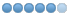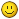# Problem writing out a 'function' expression.

• grey1beard
• Offline
• Elite Member
•07 Jun 2013 00:04 #35351
I'm trying to write a line that sends the cutter in the Z direction by an amount that is a funcion of the current X position.
The function would be Z = -[SQRT[90000+[X^2]] -300] .
If I delete the [X^2], and substitute a suitable integer, it works, but the [X^2] or [X*X] each throw up the same "unknown word where unary operation could be".
This happens even if I omit the brackets.
How do I write X squared, please ?

John

PS this is dropped into a loop instruction, where the value of X decreases each time in a constant amount, but the value of z is dependant on the absolute position of X at the start of the loop, the end result being a concave dish.
(But perhaps you didn't need to know that• grey1beard
• Offline
• Elite Member
•07 Jun 2013 04:13 #35369
Managed to solve it by two hours trial and errorg01 z[274.955-SQRT[90000-[#2]*[#2]]] F#3 is the crucial line.

John

(Dish plot with 120mm diameter and 300mm radius of curvature)
#2= -120
#3= 300

G17 G21 G40 G49 G54 G80 G90 G94

g00 x y z
g00 z

o101 while [#2 lt -2]
g3 I[#2] F#3 ;circular cut
g91
g01 x[-3]F#3 ;step over
g90
g01 z[274.955-SQRT[90000-[#2]*[#2]]] F#3 ;lower cutter
#2= [#2+3]
o101 endwhile

m30

07 Jun 2013 06:46 #35379 by BigJohnT
John,

Your having too much fun now!

JT

07 Jun 2013 21:28 #35411 by andypugh

I'm trying to write a line that sends the cutter in the Z direction by an amount that is a funcion of the current X position.
The function would be Z = -[SQRT[90000+[X^2]] -300] .
If I delete the [X^2], and substitute a suitable integer, it works, but the [X^2] or [X*X] each throw up the same "unknown word where unary operation could be".

The problem is that "X" does not mean "the position of X" it is a command, meaning "move X to here".

To use the current X position in a calculation you can use #5420
www.linuxcnc.org/docs/html/gcode/overview.html#sec:parameters

G1 Z [300 - sqrt[9000 + [#5420 ** 2]]]

(Exponentiation in LinuxCNC G-code is ** )

• grey1beard
• Offline
• Elite Member
•11 Jun 2013 00:00 #35495
Hi Andy.
I suspect we might be at cross purposes here, but thanks anyway.
I successfully cut the profile I needed, at least third time lucky, when I decided I didn't need a dish, but a dome ! (Just multiplied Z by -1 in the crucial line)

Now got a new problem, so I've started another thread.

Regards
John

PS I often don't get notifications of replies, even though the box is checked ?)